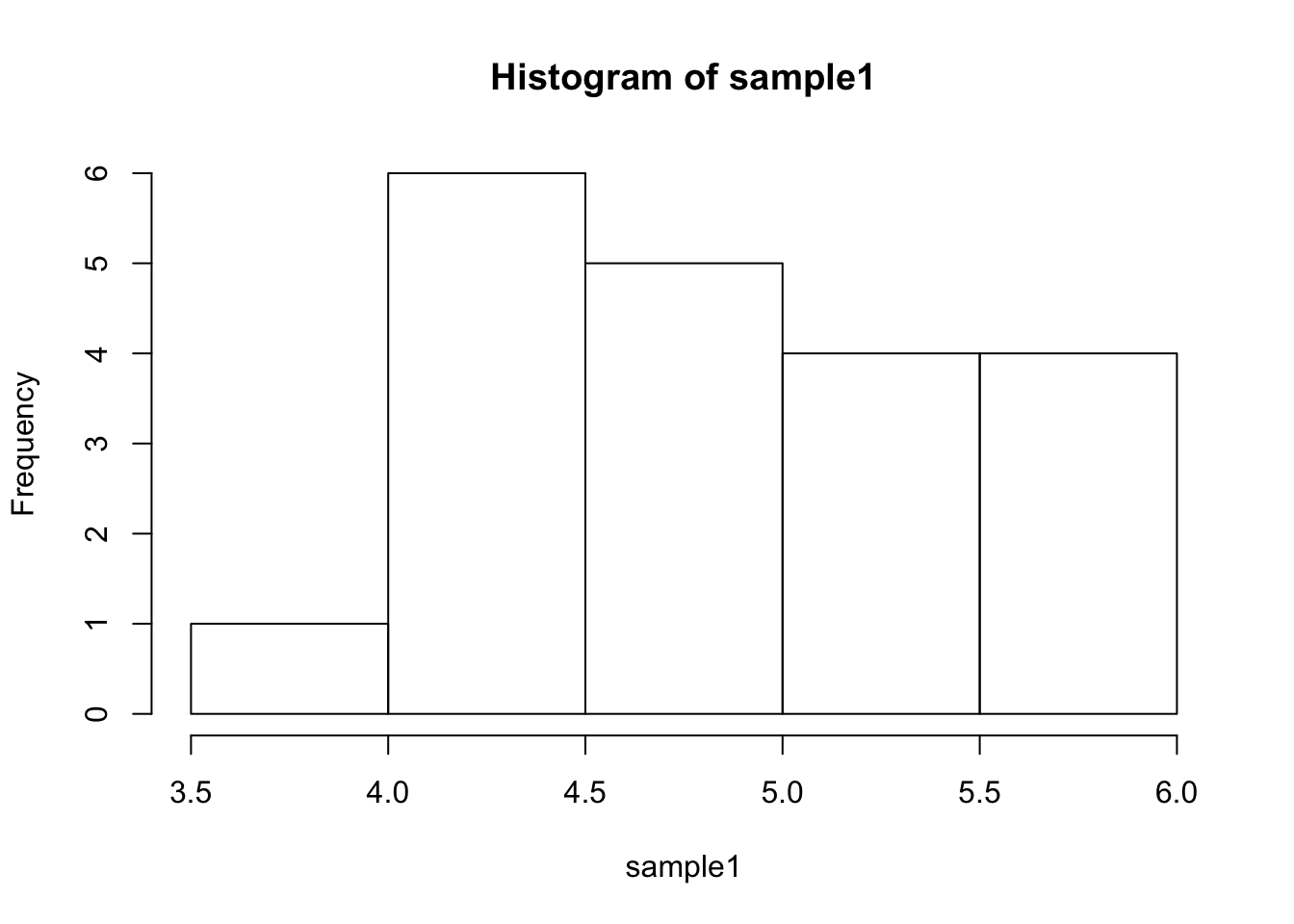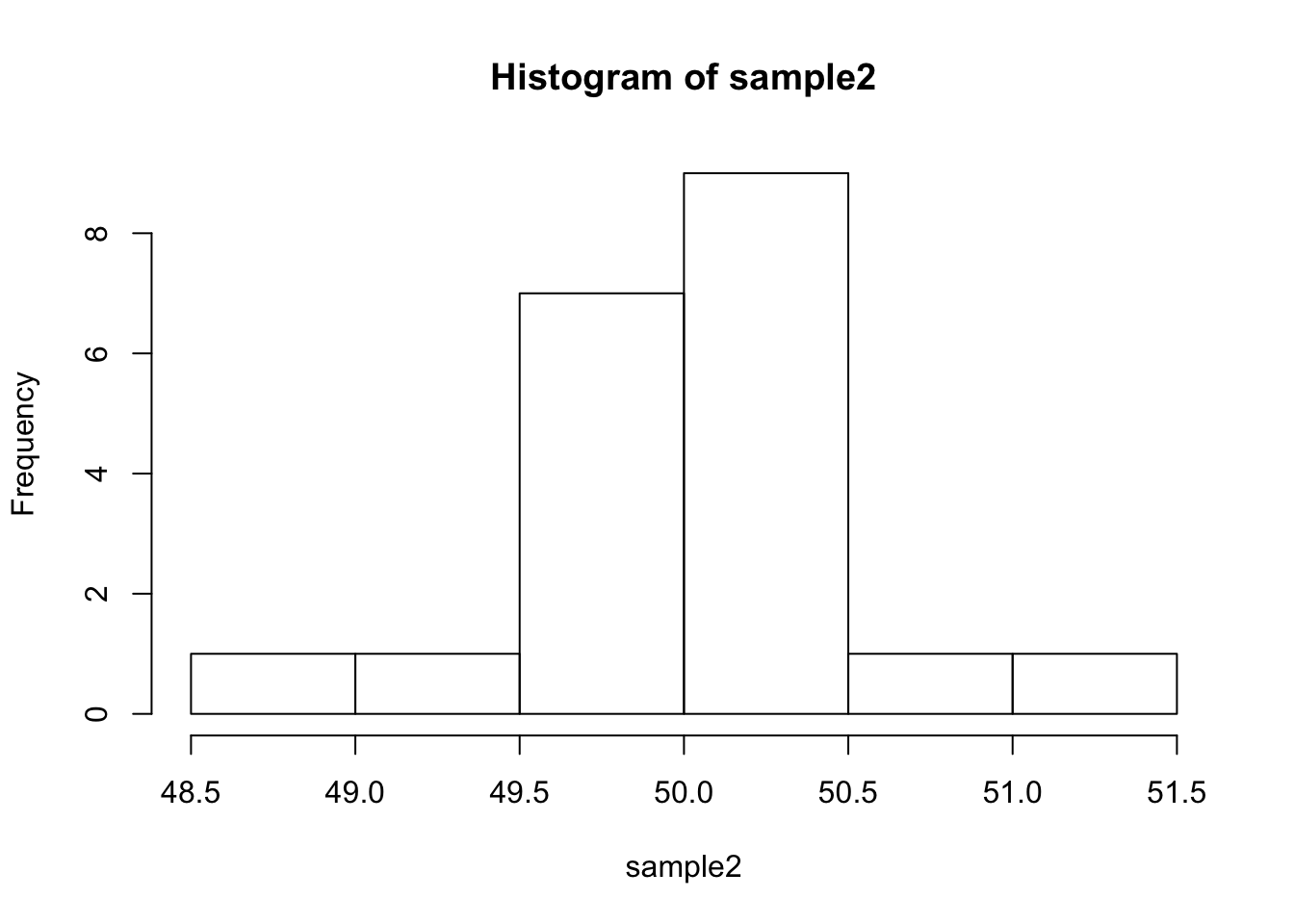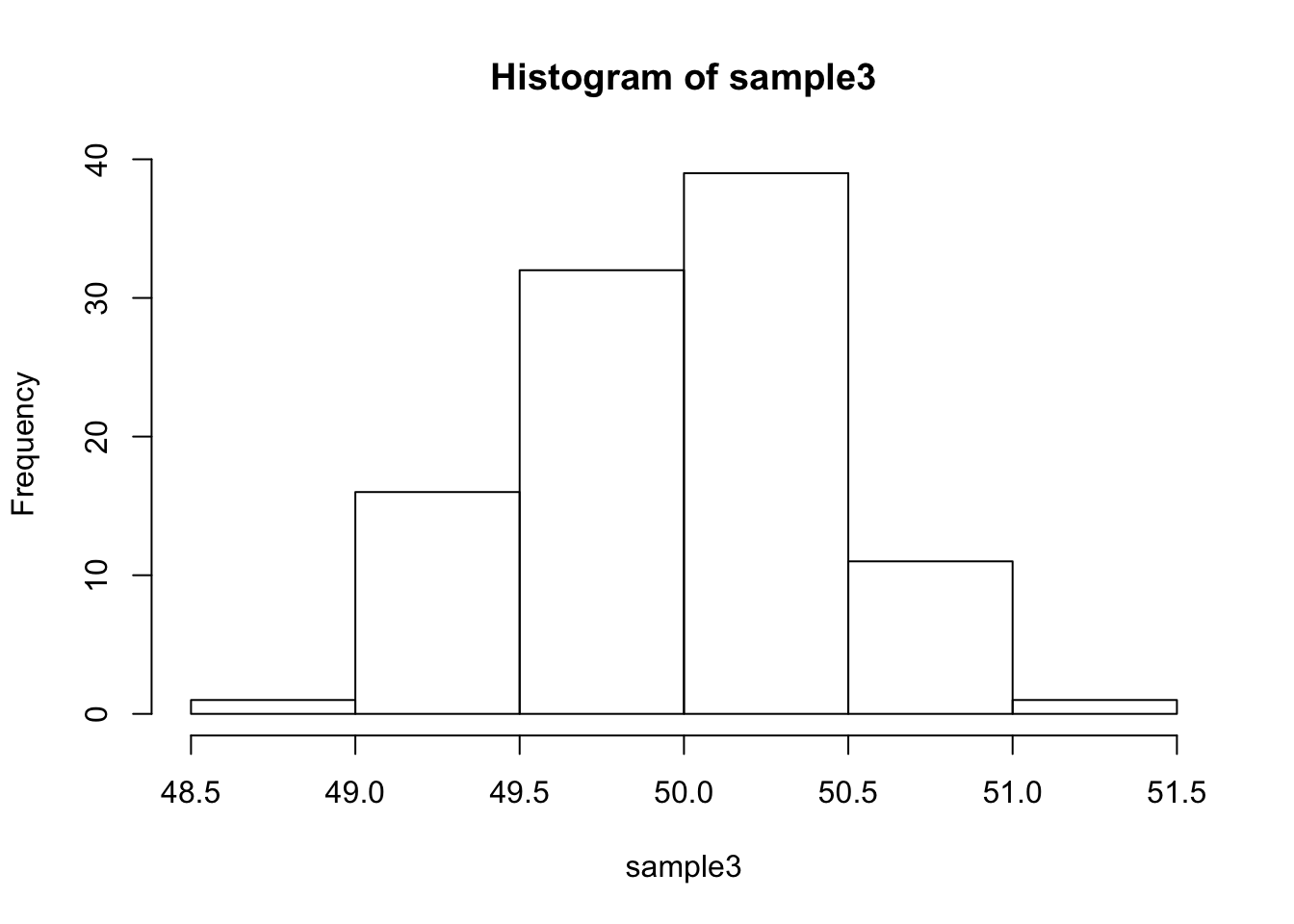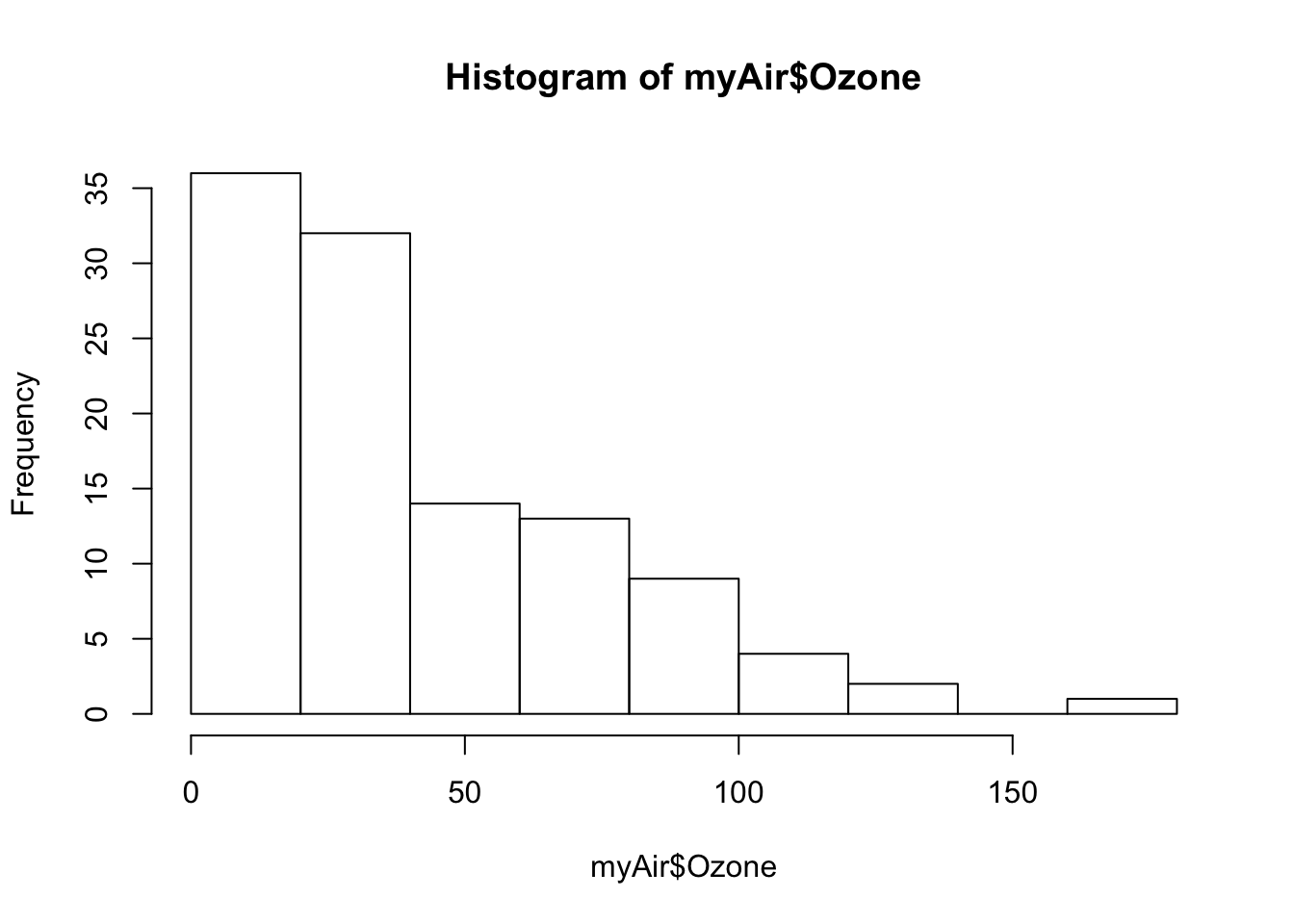## Step 1: Write a summarizing function to understand the distribution of a vector

``````#install.packages("moments")
library(moments)

# 1. create a function called "printVecInfo" that take a vector as input
printVecInfo <- function(vector)
{
# Print output to the screen. Text inside quotation marks would be printed directly.
# "\n" represents insert a new line in the test at this point.
cat("mean:", mean(vector), "\n")
cat("median:", median(vector), "\n")
cat("min:", min(vector), " max", max(vector), "\n")
cat("sd:", sd(vector), "\n")
cat("quantile(0.05-0.95):", quantile(vector, probs=0.05),"--",quantile(vector, probs=0.95), "\n")
cat("skewness:", skewness(vector))
}

# 3. test the function
printVecInfo(c(1,2,3,4,5,6,7,8,9,10,50))``````
``````## mean: 9.545455
## median: 6
## min: 1  max 50
## sd: 13.72125
## quantile(0.05-0.95): 1.5 -- 30
## skewness: 2.620396``````

## Step 2 Creating Samples in a Jar

``````# 4. Create a variable ‘jar’ that has 50 red and 50 blue marbles
# Replicate the string "red" for 50 times and store them in the vector "redMarble"
redMarble <- rep("red", 50)
# Replicate the string "blue" for 50 times and store them in the vector "blueMarble"
blueMarble <- rep("blue", 50)
# put redMarble and blueMarble together in the variable "jar"
jar <- c(redMarble, blueMarble)

# 5. Confirm there are 50 reds by summing the samples that are red
# search for "red" in vector "jar" and get the length (count how many)
length(grep("red",jar))``````
``##  50``
`````` # 6. Sample 10 ‘marbles’ from the jar. How many are red? What was the percentage of red marbles?
# sample 10 marbles from the jar
jarSample <- sample(jar, size=10, replace=TRUE)
# count how many "marbles" are red
numRed <- length(grep("red", jarSample))
# calculate the percentage of red marbles
numRed/length(jarSample)``````
``##  0.4``
``````# 7. Do the sampling 20 times, using the ‘replicate’ command.
# First, sample 10 marbles and count how many "red" there are in this sample;
# Second, repeat the first process 10 times and calculate the mean of how many "reds" there are in these samples;
# Last, repeat the all above process 20 times to get a list of 20 mean numbers.

sample1 <- replicate(20,mean(replicate(10,length(grep("red",sample(jar,size=10,replace=TRUE))))),simplify = TRUE)

printVecInfo(sample1)``````
``````## mean: 4.895
## median: 4.85
## min: 3.9  max 5.7
## sd: 0.5623962
## quantile(0.05-0.95): 4.09 -- 5.605
## skewness: -0.12958``````
`` hist(sample1)```````` # 8. Repeat #7, but this time, sample the jar 100 times. You should get 20 numbers.
# First, sample 100 marbles and count how many "red" there are in this sample;
# Second, repeat the first process 100 times and calculate the mean of how many "reds" there are in these samples;
# Last, repeat the all above process 20 times to get a list of 20 mean numbers.
sample2 <- replicate(20, mean(replicate(100,length(grep("red",sample(jar,size=100,replace=TRUE))))),simplify = TRUE)
printVecInfo(sample2)``````
``````## mean: 50.0185
## median: 50.07
## min: 48.84  max 51.19
## sd: 0.4799043
## quantile(0.05-0.95): 49.315 -- 50.7245
## skewness: -0.04029061``````
`` hist(sample2)```````` # 9. Repeat #8, but this time, replicate the sampling 100 times. You should get 100 numbers.
# repeat # 8, but in the last procedure, repeat the all process 100 times to get a list of 100 mean numbers.
sample3 <- replicate(100, mean(replicate(100,length(grep("red",sample(jar,size=100,replace=TRUE))))),simplify = TRUE)
printVecInfo(sample3)``````
``````## mean: 49.982
## median: 50.015
## min: 48.78  max 51.08
## sd: 0.4637942
## quantile(0.05-0.95): 49.2185 -- 50.76
## skewness: -0.0282589``````
`` hist(sample3)``## Step 3: Explore the airquality dataset

``````# 10. Store the ‘airquality’ dataset into a temporary variable "myAir"
myAir <- airquality

# 11. clean the dataset
myAir <- na.omit(myAir)

# 12. Explore Ozone, Wind and Temp
# Do a ‘printVecInfo’ on each variable
printVecInfo(myAir\$Ozone)``````
``````## mean: 42.0991
## median: 31
## min: 1  max 168
## sd: 33.27597
## quantile(0.05-0.95): 8.5 -- 109
## skewness: 1.248104``````
`` printVecInfo(myAir\$Wind)``
``````## mean: 9.93964
## median: 9.7
## min: 2.3  max 20.7
## sd: 3.557713
## quantile(0.05-0.95): 4.6 -- 15.5
## skewness: 0.4556414``````
`` printVecInfo(myAir\$Temp)``
``````## mean: 77.79279
## median: 79
## min: 57  max 97
## sd: 9.529969
## quantile(0.05-0.95): 61 -- 92.5
## skewness: -0.2250959``````
`````` # generate a histogram for each variable
hist(myAir\$Ozone)```````` hist(myAir\$Wind)``